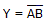# Electronics - Logic Circuit Simplification

## Why Electronics Logic Circuit Simplification?

In this section you can learn and practice Electronics Questions based on "Logic Circuit Simplification" and improve your skills in order to face the interview, competitive examination and various entrance test (CAT, GATE, GRE, MAT, Bank Exam, Railway Exam etc.) with full confidence.

## Where can I get Electronics Logic Circuit Simplification questions and answers with explanation?

IndiaBIX provides you lots of fully solved Electronics (Logic Circuit Simplification) questions and answers with Explanation. Solved examples with detailed answer description, explanation are given and it would be easy to understand. All students, freshers can download Electronics Logic Circuit Simplification quiz questions with answers as PDF files and eBooks.

## Where can I get Electronics Logic Circuit Simplification Interview Questions and Answers (objective type, multiple choice)?

Here you can find objective type Electronics Logic Circuit Simplification questions and answers for interview and entrance examination. Multiple choice and true or false type questions are also provided.

## How to solve Electronics Logic Circuit Simplification problems?

You can easily solve all kind of Electronics questions based on Logic Circuit Simplification by practicing the objective type exercises given below, also get shortcut methods to solve Electronics Logic Circuit Simplification problems.

### Exercise :: Logic Circuit Simplification - General Questions

1.

Which statement below best describes a Karnaugh map?

 A. It is simply a rearranged truth table. B. The Karnaugh map eliminates the need for using NAND and NOR gates. C. Variable complements can be eliminated by using Karnaugh maps. D. A Karnaugh map can be used to replace Boolean rules.

Explanation:

No answer description available for this question. Let us discuss.

2.

Which of the examples below expresses the commutative law of multiplication?

 A. A + B = B + A B. A • B = B + A C. A • (B • C) = (A • B) • C D. A • B = B • A

Explanation:

No answer description available for this question. Let us discuss.

3.

The Boolean expressionis logically equivalent to what single gate?

 A. NAND B. NOR C. AND D. OR

Explanation:

No answer description available for this question. Let us discuss.

4.

The observation that a bubbled input OR gate is interchangeable with a bubbled output AND gate is referred to as:

 A. a Karnaugh map B. DeMorgan's second theorem C. the commutative law of addition D. the associative law of multiplication

Explanation:

No answer description available for this question. Let us discuss.

5.

The systematic reduction of logic circuits is accomplished by:

 A. symbolic reduction B. TTL logic C. using Boolean algebra D. using a truth table